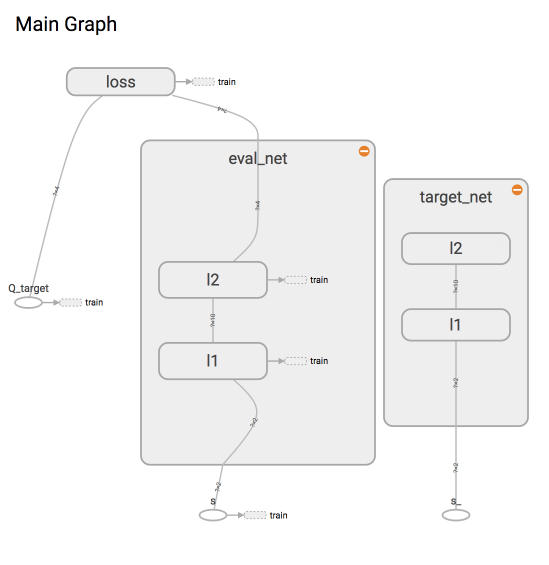# DQN 神经网络 (Tensorflow)

## 两个神经网络 ¶## 神经网络结构 ¶

class DeepQNetwork:
# 建立神经网络
def _build_net(self):


## 创建两个网络 ¶

class DeepQNetwork:
def _build_net(self):
# -------------- 创建 eval 神经网络, 及时提升参数 --------------
self.s = tf.placeholder(tf.float32, [None, self.n_features], name='s')  # 用来接收 observation
self.q_target = tf.placeholder(tf.float32, [None, self.n_actions], name='Q_target') # 用来接收 q_target 的值, 这个之后会通过计算得到
with tf.variable_scope('eval_net'):
# c_names(collections_names) 是在更新 target_net 参数时会用到
c_names, n_l1, w_initializer, b_initializer = \
['eval_net_params', tf.GraphKeys.GLOBAL_VARIABLES], 10, \
tf.random_normal_initializer(0., 0.3), tf.constant_initializer(0.1)  # config of layers

# eval_net 的第一层. collections 是在更新 target_net 参数时会用到
with tf.variable_scope('l1'):
w1 = tf.get_variable('w1', [self.n_features, n_l1], initializer=w_initializer, collections=c_names)
b1 = tf.get_variable('b1', [1, n_l1], initializer=b_initializer, collections=c_names)
l1 = tf.nn.relu(tf.matmul(self.s, w1) + b1)

# eval_net 的第二层. collections 是在更新 target_net 参数时会用到
with tf.variable_scope('l2'):
w2 = tf.get_variable('w2', [n_l1, self.n_actions], initializer=w_initializer, collections=c_names)
b2 = tf.get_variable('b2', [1, self.n_actions], initializer=b_initializer, collections=c_names)
self.q_eval = tf.matmul(l1, w2) + b2

with tf.variable_scope('loss'): # 求误差
self.loss = tf.reduce_mean(tf.squared_difference(self.q_target, self.q_eval))
with tf.variable_scope('train'):    # 梯度下降
self._train_op = tf.train.RMSPropOptimizer(self.lr).minimize(self.loss)

# ---------------- 创建 target 神经网络, 提供 target Q ---------------------
self.s_ = tf.placeholder(tf.float32, [None, self.n_features], name='s_')    # 接收下个 observation
with tf.variable_scope('target_net'):
# c_names(collections_names) 是在更新 target_net 参数时会用到
c_names = ['target_net_params', tf.GraphKeys.GLOBAL_VARIABLES]

# target_net 的第一层. collections 是在更新 target_net 参数时会用到
with tf.variable_scope('l1'):
w1 = tf.get_variable('w1', [self.n_features, n_l1], initializer=w_initializer, collections=c_names)
b1 = tf.get_variable('b1', [1, n_l1], initializer=b_initializer, collections=c_names)
l1 = tf.nn.relu(tf.matmul(self.s_, w1) + b1)

# target_net 的第二层. collections 是在更新 target_net 参数时会用到
with tf.variable_scope('l2'):
w2 = tf.get_variable('w2', [n_l1, self.n_actions], initializer=w_initializer, collections=c_names)
b2 = tf.get_variable('b2', [1, self.n_actions], initializer=b_initializer, collections=c_names)
self.q_next = tf.matmul(l1, w2) + b2